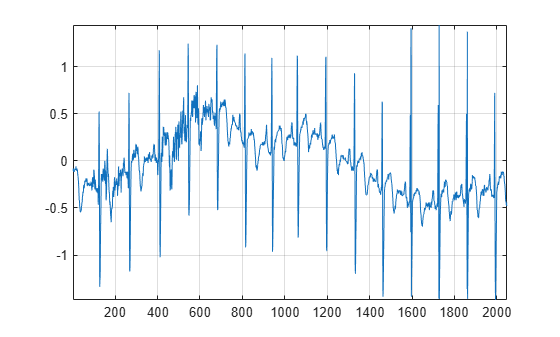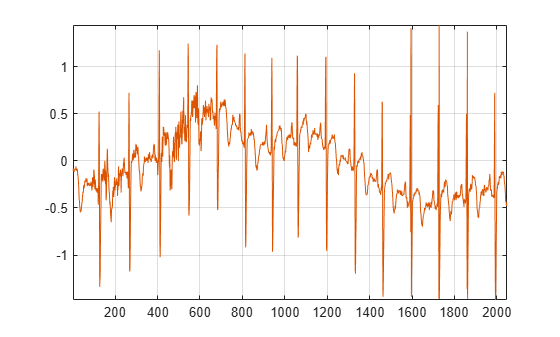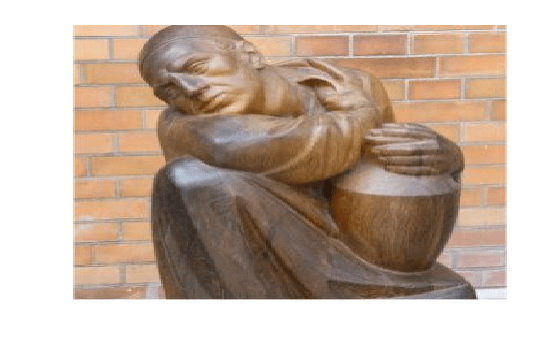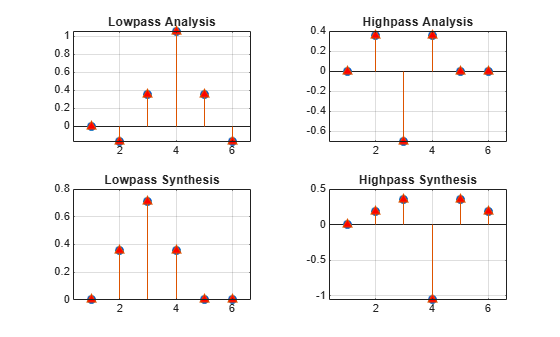# Construct Perfect Reconstruction Biorthogonal Filter Bank

This example shows how to take an expression of a biorthogonal filter pair and construct lowpass and highpass filters to produce a perfect reconstruction (PR) biorthogonal wavelet filter bank.

The LeGall 5/3 filter is the wavelet used in JPEG2000 for lossless image compression. The lowpass (scaling) filters for the LeGall 5/3 wavelet have five and three nonzero coefficients respectively. The expressions for these two filters are:

`${H}_{0}\left(z\right)=1/8\left(-{z}^{2}+2z+6+2{z}^{-1}-{z}^{-2}\right)$`

`${H}_{1}\left(z\right)=1/2\left(z+2+{z}^{-1}\right)$`

Create these filters.

```H0 = 1/8*[-1 2 6 2 -1]; H1 = 1/2*[1 2 1];```

Many of the discrete wavelet and wavelet packet transforms in Wavelet Toolbox™ rely on the filters being both even-length and equal in length in order to produce the perfect reconstruction filter bank associated with these transforms. These transforms also require a specific normalization of the coefficients in the filters for the algorithms to produce a PR filter bank. Use the `biorfilt` function on the lowpass prototype functions to produce the PR wavelet filter bank.

`[LoD,HiD,LoR,HiR] = biorfilt(H0,H1);`

Use the `isbiorthwfb` function to confirm the four filters jointly satisfy the necessary and sufficient conditions to be a two-channel biorthogonal PR wavelet filter bank.

`[tf,checks] = isbiorthwfb(LoR,LoD,HiR,HiD)`
```tf = logical 1 ```
```checks=7×3 table Pass-Fail Maximum Error Test Tolerance _________ _____________ ______________ Dual filter lengths correct pass 0 0 Filter sums pass 2.7756e-17 1.4901e-08 Zero lag lowpass dual filter cross-correlation pass 2.2204e-16 1.4901e-08 Zero lag highpass dual filter cross-correlation pass 2.2204e-16 1.4901e-08 Even lag lowpass dual filter cross-correlation pass 0 1.4901e-08 Even lag highpass dual filter cross-correlation pass 0 1.4901e-08 Even lag lowpass-highpass cross-correlation pass 1.3878e-17 1.4901e-08 ```

Now you can use these filters in discrete wavelet and wavelet packet transforms. To demonstrate perfect reconstruction, load and plot an ECG signal.

```load wecg plot(wecg) axis tight grid on```Obtain the discrete wavelet packet transform of the ECG signal using the LeGall 5/3 filter set.

`[wpt,L] = dwpt(wecg,LoD,HiD);`

Now use the reconstruction (synthesis) filters to reconstruct the signal and demonstrate perfect reconstruction.

```xrec = idwpt(wpt,L,LoR,HiR); plot([wecg xrec]) axis tight grid on````norm(wecg-xrec,"inf")`
```ans = 3.3307e-15 ```

You can also use this filter bank in the 1-D and 2-D discrete wavelet transforms. Read and plot an image.

```im = imread('woodsculp256.jpg'); image(im) axis off```Obtain the 2-D wavelet transform using the LeGall 5/3 analysis filters.

`[C,S] = wavedec2(im,3,LoD,HiD);`

Reconstruct the image using the synthesis filters.

`imrec = waverec2(C,S,LoR,HiR);`

Demonstrate perfect reconstruction.

`norm(double(im)-imrec,"fro")`
```ans = 7.2465e-11 ```

The LeGall 5/3 filter is equivalent to the built-in `bior2.2` wavelet in the toolbox. Confirm the LeGall 5/3 filters are equal to the filters associated with the `bior2.2` wavelet.

```[LD,HD,LR,HR] = wfilters("bior2.2"); tiledlayout(2,2) nexttile hl = stem([LD' LoD']); hl(1).MarkerFaceColor = [0 0 1]; hl(1).Marker = "o"; hl(2).MarkerFaceColor = [1 0 0]; hl(2).Marker = "^"; grid on title("Lowpass Analysis") nexttile hl = stem([HD' HiD']); hl(1).MarkerFaceColor = [0 0 1]; hl(1).Marker = "o"; hl(2).MarkerFaceColor = [1 0 0]; hl(2).Marker = "^"; grid on title("Highpass Analysis") nexttile hl = stem([LR' LoR']); hl(1).MarkerFaceColor = [0 0 1]; hl(1).Marker = "o"; hl(2).MarkerFaceColor = [1 0 0]; hl(2).Marker = "^"; grid on title("Lowpass Synthesis") nexttile hl = stem([HR' HiR']); hl(1).MarkerFaceColor = [0 0 1]; hl(1).Marker = "o"; hl(2).MarkerFaceColor = [1 0 0]; hl(2).Marker = "^"; grid on title("Highpass Synthesis")```